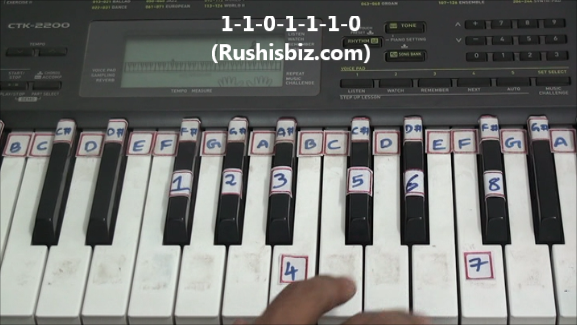HINDI - A B C D E F G H I J K L M N O P Q R S T U V W X Y Z

TAMIL - A B C D E F G H I J K L M N O P Q R S T U V W X Y Z

TELUGU - A B C D E F G H I J K L M N O P Q R S T U V W X Y Z

# “F# /Gb” Major Scale Positions – Piano Tutorials

Notes:  F#-1, G#-2, A#-3, B-4, C#-5, D#-6, F-7, F#-8

Since it is a ‘F#’ major scale obviously ‘F#’ will be first note, how to find the remaining notes ?

The simple formula which is used for any major scale:

One- One- Zero- One-One- One-Zero  (1-1-0-1-1-1-0)

What do you mean by Ones and Zeros ?

One’s (whole step) – If there is a one key between two notes, then the interval of those two notes is One.

Eg: from F# to G# the interval is “one key”

Zero’s (half step) – If there are no keys or zero keys between two notes, then the interval of those two notes is Zero

Eg: from A# to B the interval is “zero” (Immediate keys)‘F#’ Major Scale Positions – Video Tutorials